BLOG

# Pressure units and pressure unit conversion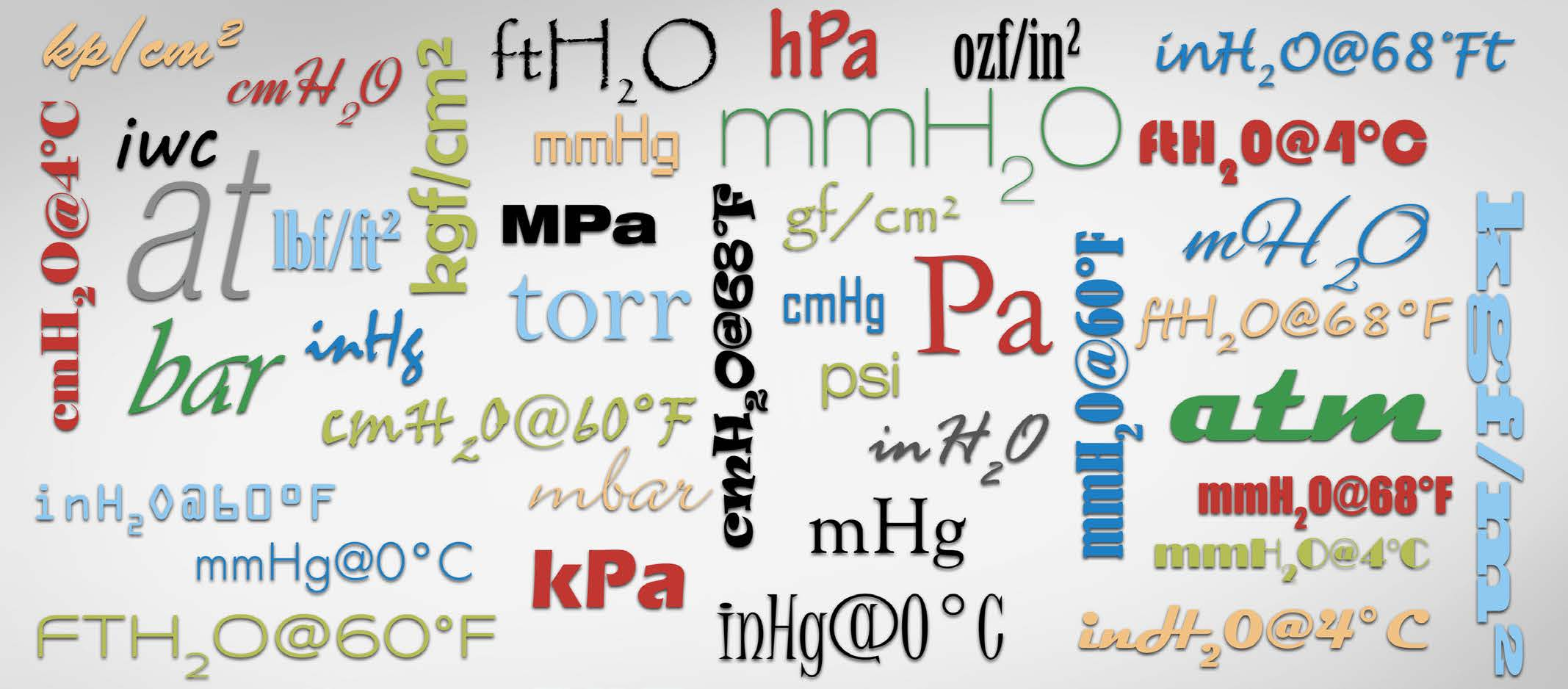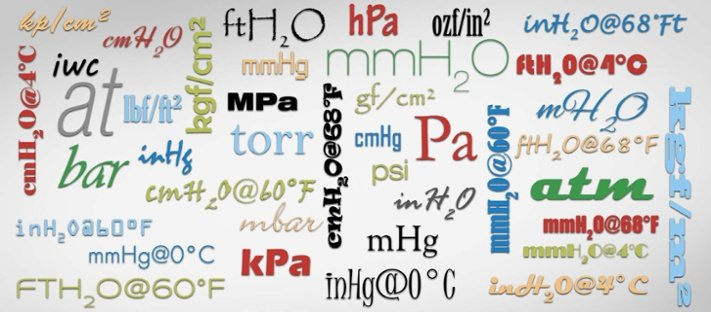## It’s a jungle out there!

There are a lot of different pressure units in use around the world and sometimes this can be very confusing and may cause dangerous misunderstandings.

In this blog post, I will discuss the basics of different pressure units and different pressure unit families.

If you just want to convert between different pressure units, please check out our handy free pressure unit converter

Now that we are talking about pressure, let's start with a video on how to calibrate a pressure transmitter:

### What is Pressure?

When I talk about pressure in this post, it does not refer to the stress you may be suffering in your work, but to the physical quantity. It is good to first take a quick look at the definition of pressure, this will also help to better understand some of the pressure units.

If you remember the studies of physics in school … as most of us don’t remember… a short reminder is in order: pressure is defined as force per area perpendicular to the surface. That is often presented as formula p = F/A. The pressure is indicated with the letter “p”, although the capital letter “P” can also be seen being used on some occasions.

So what does this force per area mean in practice? It means that there is a certain force affecting a specified area. When we look at force, it is specified as being Mass x Gravity. As there are so many different engineering units used for both mass and area, the number of combinations of these is huge. Plus there are also a lot of pressure units that do not directly have the mass and area in their names, although it often is in their definition.

It is good to notice that in practice the “force” is not always included in the pressure unit names. For example, pressure unit kilogram-force per square centimeter should be indicated as kgf/cm², but often it is indicated just as kg/cm² without the “f ”. Similarly, pound-force per square inch (pfsi) is normally indicated as pounds per square inch (psi).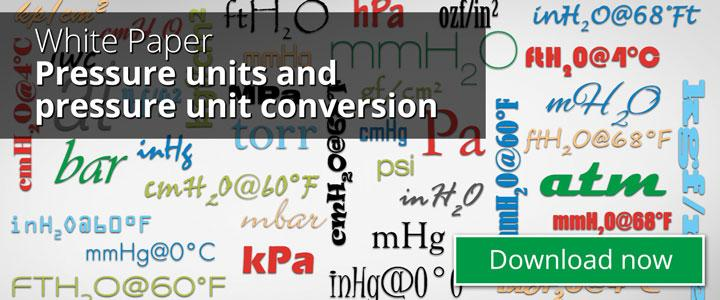### International System of Units (SI system) / Metric

Let’s start to look at the pressure units by looking at the SI system, which is the International System of Units, derived from the metric system. Now that I mentioned the metric system, I can already see some of you taking a step back… but please stay with me!

SI system is the world’s most widely used system of measurement. It was published in 1960 but has a very long history even before that.

## What is Pascal?

Pascal (Pa) is the standard unit of pressure used to measure the amount of force exerted per unit area. It is named after Blaise Pascal, a French mathematician, and physicist. One pascal is a relatively small amount of pressure, and it is equivalent to the force of one newton per square meter. However, for practical purposes, gas pressures are often measured in kilopascals (kPa), which is equal to 1000 pascals.

Pascal is the SI unit of pressure, and is the basic pressure unit in the SI (the International System of Units) system.

Pascal is not that old base unit is SI system, as it was adopted in 1971.

Pascal is a so-called “SI derived unit” of pressure, as it is derived from the base units specified in the SI system.

The definition of one Pascal is the pressure of one Newton per square meter.
A Newton is a force needed to accelerate one kilogram of mass at the rate of one meter per second.

So, we can say one Newton is one kgm/s².

To say Pascal (Pa) in a formula: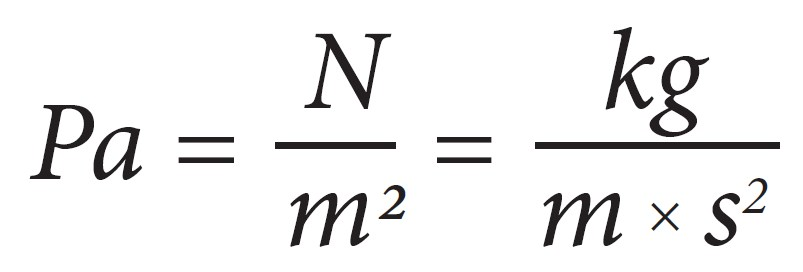Pascal is a very small pressure unit and is often used with different prefixes. Common multiples include hectopascal (1 hPa = 100 Pa), kilopascal (1 kPa = 1 000 Pa) and megapascal (1 MPa = 1 000 000 Pa).

The standard atmospheric pressure is over one hundred thousand Pascals, to be exact it is 101 325 Pa, or 101.325 kPa.

In our (Beamex) accredited calibration laboratory we document all pressure calibrations in Pascals in calibration certificates (with suitable multiples).

We commonly see Pascal pressure unit being used in cleanroom differential pressure measurement transmitters or gauges, as the difference between different rooms/areas is commonly only tens of Pascals.
Calibrating these very low-pressure instruments is a challenge of its own.

Out of Pascal’s definition, the kg force can be replaced with different units like g (gram) force, and the meter can be replaced with centimeters or millimeters. By doing that, we get many other combinations or pressure units, such as kgf/m², gf/m², kgf/cm², gf/cm², kgf/mm², gf/mm², just to list a few.

The unit “bar” is still often used in some areas. It is based on the metric system but is not part of SI system. Bar being 100 000 times Pascal (100 times kPa) it is anyhow easy to convert. In some areas (like NIST in the USA) the bar is not recommended to be used widely.

And like for all pressure units, SI or not SI, we can use the common prefixes/coefficients in front of them, most commonly used are milli (1/100), centi (1/10), hecto (100), kilo (1000) and mega (1000000). To list a few examples, that already gives us different Pa versions, all being commonly used: Pa, kPa, hPa, MPa. The unit bar is most commonly used without prefix or with prefix milli: bar, mbar.

But taking all mass units and combining those with all area units from the SI system, we get many combinations.

Although the SI system is used in most countries, there are still a lot of other pressure units also being used. So let’s take a look at those next.

### Imperial units

In countries using the Imperial system (like the USA and UK), the engineering units used both for mass and area are different than with the SI system. Therefore this also creates a whole new set of pressure units. Mass is being measured commonly in pounds or ounces, and area and distance with inches or feet.
So some pressure units derived from these are lbf/ft², psi, ozf/in², iwc, inH2O, ftH2O.

In the United States, the most common pressure unit is pounds per square inch (psi). For process industries, a common unit is also inches of water (inH2O), which is derived from level measurement and the historical measurements of pressure differences with water in a column.

### Liquid column units

The older pressure measurement devices were often made by using liquid in a transparent U-tube. If the pressure in both ends of the tube is the same, the liquid level on both sides is on the same level. But if there is a difference in the pressures, then there is a difference in the liquid levels. The level difference is linearly proportional to the pressure difference. In practice, you can leave one side of the tube open to the room’s atmospheric pressure and connect the pressure to be measured to the other side. As referred to the current atmospheric pressure, it is a gauge pressure type being measured.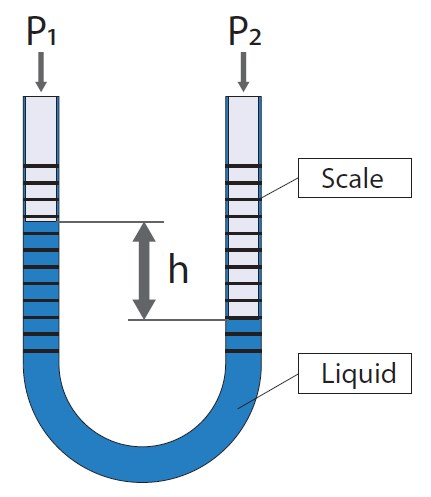The pressure scale is marked in the tube so you read the pressure by reading the difference in liquid levels. When pressure is applied it will change the liquid level and we can read the value. This sounds very simple, no electronics and no wearing parts, so what could possibly go wrong… well, let’s see about that.

The most commonly used liquid in the column was obviously water. But in order to be able to measure higher pressure with a smaller U-tube, heavier liquids were needed. One such liquid is mercury (Hg) as it is much heavier than water (13.6 times heavier). When you use the heavier liquid you don’t need to have that long column to measure higher pressure, so you can make a smaller and more convenient size column. For example, blood pressure was earlier (still sometimes is) measured with a mercury column. Mercury is mainly used because a water column for the same pressure range would be so long it would not be practical to use it in a normal room, as the water column is about 13.6 times longer than the mercury column. As a result of this, even today the pressure unit that blood pressure is typically expressed is a millimeter of mercury (mmHg).

A common industrial application for use of liquid column pressure units is to measure the liquid level in a tank. For example, if you have a water tank that is 20 feet (or 6 meters) high and you want to measure the water level in that tank, it sounds pretty logical to install a pressure indicator with a scale 0 to 20 feet of water, as that would tell straight what the water level is (13 feet in the example picture).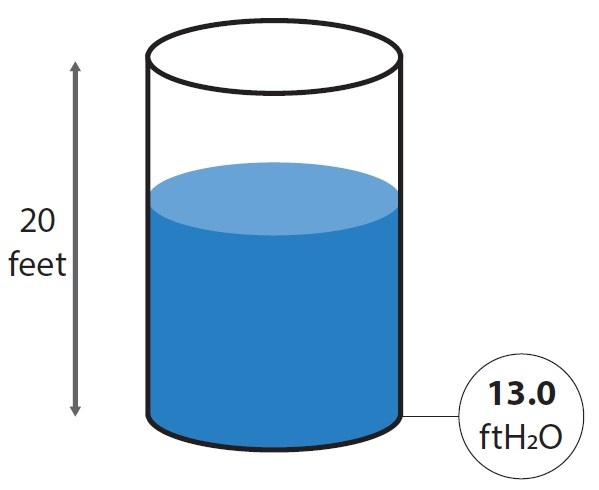Back to the water column: It is clear that when the length indication was made to a U column, many different length units have been used, both metric and non-metric. This has generated many different pressure units.

Although a liquid column sounds very simple, it is important to remember that the weight of the liquid depends on the local gravity, so if you calibrate the column in one place and take it to another (distant, different elevation) place, it may not be measuring correctly anymore. So gravity correction is needed to be precise.

Also, the temperature of the liquid affects the density of the liquid and that also affects slightly the readings of a U-tube. There are various different liquid column-based pressure units available, having the liquid temperature specified in the pressure unit, most commonly used temperatures are 0 °C, 4 °C, 60 °F, 68 °F. But there are also water column units, which have no indication of the water temperature. These are based on a theoretical density of water, being 1 kg/1 liter (ISO31-3, BS350). In practice, the water never has that high density. The highest density that water has is at +4 °C (39.2 °F) where it is approximately 0.999972 kg/liter. The density of water gets lower if the temperature is higher or lower than +4 °C. Temperature can have a pretty strong effect on the density, for example going from +4 °C to +30 °C changes the water density by about 0.4%.

Finally, the readability of a mechanical liquid column is typically pretty limited, so you can’t get very accurate measurements. And due to the mechanical limitations, you can’t use a U-tube for high pressure. All of these above-mentioned issues make a U-tube liquid column not very practical to use. Also, modern digital pressure measurement devices have replaced liquid columns. But many of the pressure units created in the era of liquid columns have remained and are still used today. To shortly summarize the liquid column-based pressure units:

• For the length we have many units; mm, cm, m, inch and feet.
• Then we have columns for different liquids, like water (H2O) and mercury (Hg).
• We have water column units for different density at temperatures, like 0 °C, 4 °C, 60 °F and 68 °F and for theoretical densities.

By combining all of these, we get a long list of pressure units, just to mention a few: mmH₂O, cmH₂O, mH2O, mmHg, cmHg, mHg, iwc, inH2O, ftH2O, inHg, mmH2O@4°C, mmH2O@60°F, mmH2O@68°F, cmH2O@4°C, cmH2O@60°F, cmH2O@68°F, inH2O@60°F, inH2O@68°F, inH2O@4°C, ftH2O@60°F,ftH2O@68°F, ftH2O@4°C and so on.

### Atmospheric units

For measurement of the atmospherics’ absolute pressure, dedicated pressure units have been created. One of such is the standard atmosphere (atm) which is defined being 101325 Pascal. To add confusion, there is also a technical atmosphere (at) which is pretty close, but not quite the same as atm. The technical atmosphere is one-kilogram force per square centimeter. So 1 at equals about 0.968 atm.

Another pressure unit used for measuring atmospheric absolute pressure is torr, being 1/760 of standard atmosphere. So torr is an absolute pressure unit, although that is typically not mentioned, you just need to know it, which can cause confusion. Torr was initially meant to be the same as 1 millimeter of mercury, although the later definitions show a very small difference in between. Torr is not part of the SI system.

## Cgs unit of pressure

The abbreviation “cgs” comes from the words “centimetre-gram-second”. As these words hint, the cgs system is a variation of the metric system, but instead of using the meter it uses centimeter as the unit for length, and instead of kilogram it uses gram as the unit for mass.
Different cgs mechanical units are derived from using these cgs base units.

The cgs is a pretty old system and has been mostly replaced first by the MKS (meter-kilogram-second) system which then has been replaced by the SI system. Yet, you can still sometimes run into cgs units of pressure.

The cgs base pressure unit is barye (Ba), which equals 1 dyne per square centimeter.

Dyne is the force needed to accelerate a mass of one gram to a rate of one centimeter per second per second.

As pressure unit conversion, 1 barye (Ba) equals 0.1 Pascal (Pa).

And some more…

In addition to all the above pressure units, there are still plenty
more existing…
Just to mention, for example in a Beamex MC6 calibrator, there are over 40 different pressure units, plus still a few custom units for the thrill-seekers.

Pressure unit conversions standards

If you work with pressure, you know that it is very common that pressure is indicated with a certain pressure unit and you need to convert it into another pressure unit.

Pressure units are based on standards and the conversion between units should also be based on standards. The most common standards for pressure units are:

• SI system
• ISO31-3
• ISO 80000-4:2006
• BS350
• PTB-Mitteilungen 100 3/90
• Perry’s Chemical Engineer’s Handbook, 6th ed, 1984

Pressure unit converter tool

I tried to make a conversion table between different pressure units, but that table started quickly to become a huge matrix that would not be easy for you to use at all. So instead of making a conversion table, we developed an online pressure unit converter for our website. With this converter, you can easily convert a pressure reading from one unit into other units. Please click on the link to check out the pressure unit converter.

Below is an example screenshot from the pressure unit converter, when converting 1 MPa into other units: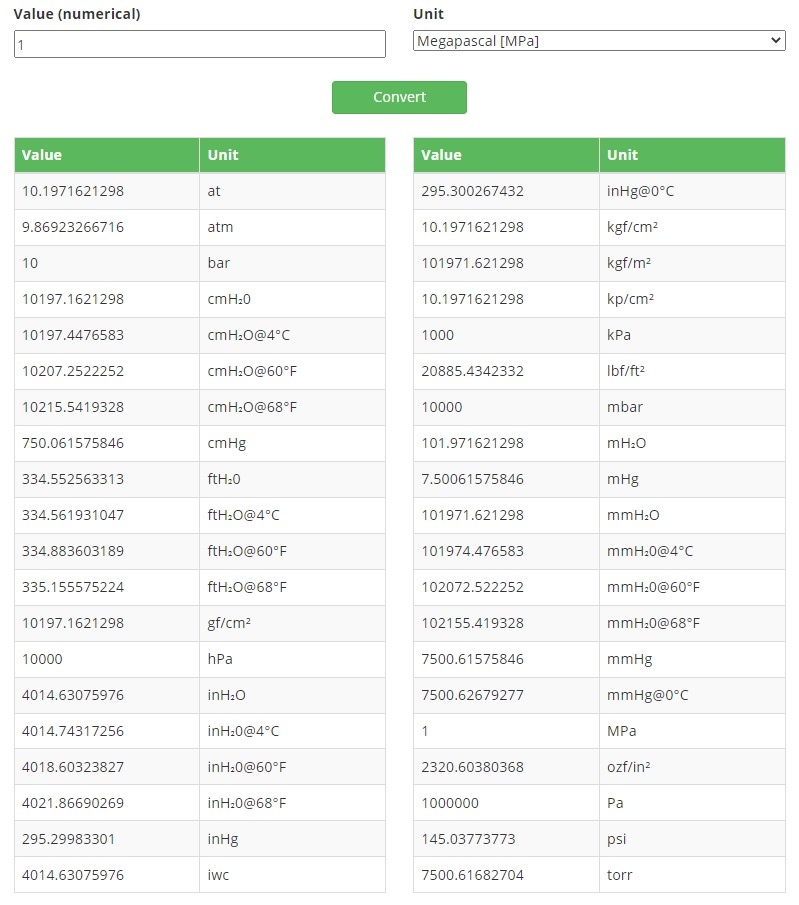Interested in printing this text or sharing it with your peers?### Beamex pressure calibration tools

Please check out our new electric pressure pump Beamex ePG: